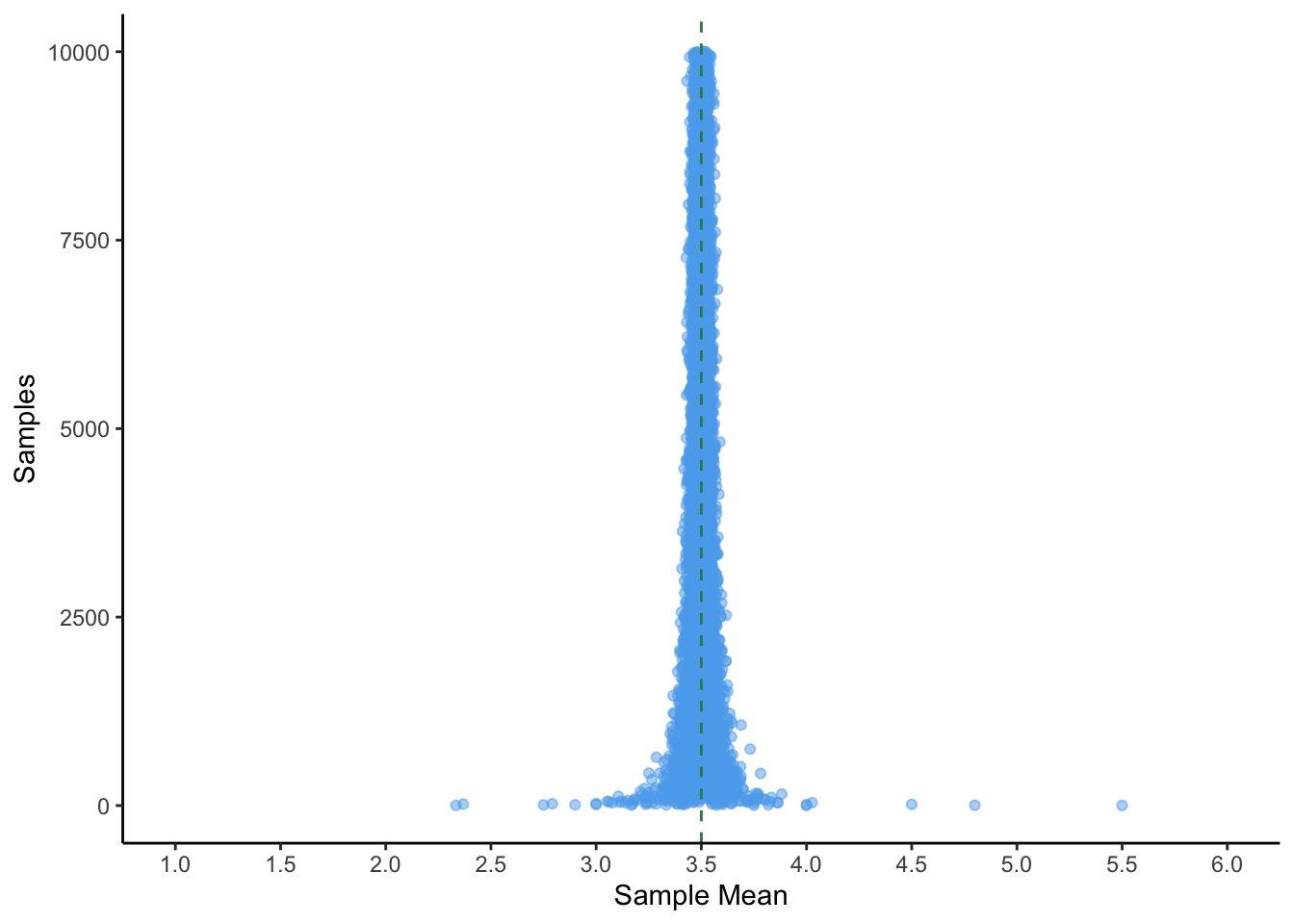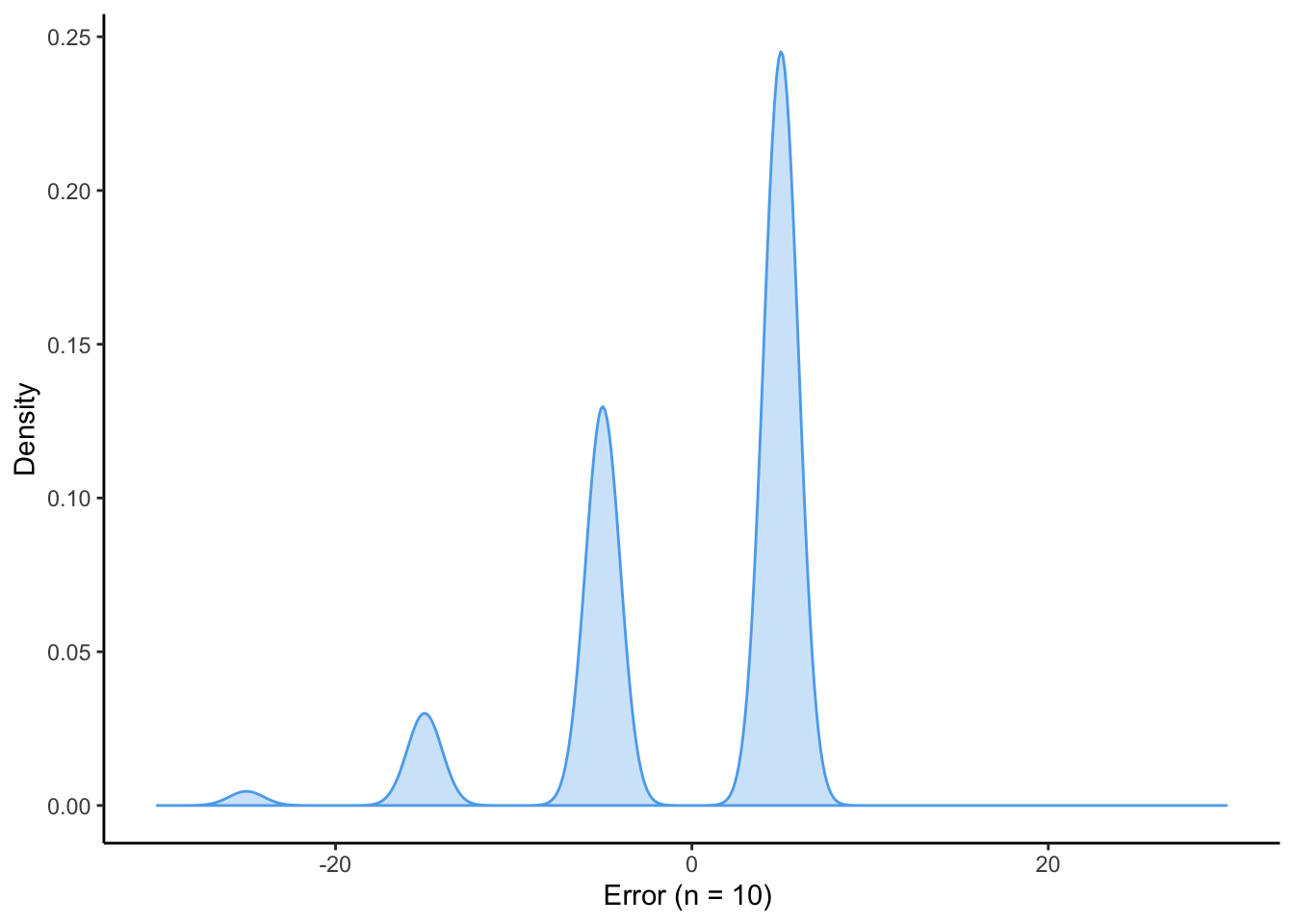### I.I.D. Random Variables

Independent and identically distrubuted

• Independent: Each draw from a random variable $$X$$ does not depend on the outcome of other draws.

$F_{X,Y}(x,y) = F_X(x) \times F_Y(y) \forall x, y$

• Identically distributed: Each random variable has the same probability distribution as the others.

$F_x(x) = F_Y(x) \forall x$

• Examples: Are they following processese I.I.D. or not?
• A sequence of fair die rolls.
• A sequence of unfair die rolls.
• Consider an urn with two balls in it, one black and one white. We reach into the urn and draw out the two balls one after the other, choosing the first one at random.
• A spin of a roulette wheel.
• Assume you have a special dice with 6 faces. If the last time the face value is 1, next time you throw it, you will still get a face value of 1 with 0.5 probability and a face value of 2,3,4,5,6 each with 0.1 probability. However, if the last time the face value is not 1, you get equal probability of each face.

• IID does not mean all events have the same probability of occuring.

### Random sampling

• A random generative process that selects one unit from a finite population $$U$$ at random, with all units having an equal probability of being selected. Under random sampling, the distribution of outcomes in the population entirely determines the probability distrubution of the random variable.
• $$E[X]$$ = population mean
• $$V[X]$$ = population variance

### Estimation

• Sample statistic: summarizes the values of random variables.
• Sample mean: The average of all of the observed values of a random variable.
• weak law of large numbers: The sample mean tends to approximate the population mean. As $$n$$ (the sample size) gets larger, the sample mean $$\bar{X}$$ becomes increasingly likely to to approximate $$E[X]$$. In other words, as the sample size grows, the sample mean under random sampling is increasingly likely to approximate the population mean.

$\bar{X} = \frac{1}{n} \sum_{i=1}^n X_i$

• Sample variance: the variance of the observed values of a random variable $$\bar{X}$$. The sampling variance decreases as $$n$$ increases.

$V[\bar{X}] = \frac{V[X]}{n}$

Example of the weak law of large numbers in action:## Estimation theory

### Some terminology

• Estimand: our quantity of interest.
• Estimator: Statistical procedure (i.e. method) used to make a guess about a parameter. This is a random variable. We use the “hat” symbol to denote an estimator.
• Estimate: the value of the estimand that results from use of a particular estimator (i.e. the value the estimator takes on).
• Asymptotics: describing the limiting behavior of a function. What happens as $$n$$ becomes very large. What are the properties of the function as $$n \rightarrow \infty$$

### Unbiasedness

• How often does an estimator give us the the right answer on average?
• Formally, the expected value of an estimator is equal to the true value of our population feature of interest (i.e. $$E[\hat{\theta}] = \theta$$)
• To measure the biasedness of an estimator: $$E[\hat{\theta}] - \theta$$

Example:

Imagine a population consisting of three units. Each unit has an associated measurement: $$Y_1$$, $$Y_2$$ and $$Y_3$$. You are interested in the average $$Y_{avg} = (Y_1 + Y_2 + Y_3)/3$$. You draw a sample of two units without replacement with equal probability and observe their measurements $${Y_a,Y_b}$$. You plan to estimate $$Y_{avg}$$ with the estimator $$\hat{Y} = \frac{(Y_a + Y_b )}{2}$$.

1. Derive the bias: $$E[\hat{Y} − Y_{avg}]$$.
2. Derive the variance: $$Var(\hat{Y})$$.
3. Derive the mean squared error: $$E[(\hat{Y} − Y_{avg})^2]$$. Hint: MSE can be written as the sum of the variance of the estimator and the squared bias of the estimator.

a.) Due to linearity of expectations and the fact that $$Y_{avg}$$ is given to be the population mean, $$E[\hat{Y} - Y_{avg}] = E[\hat{Y}] - E[Y_{avg}] = E[\hat{Y}] - Y_{avg}$$.

$E[\hat{Y} - Y_{AVG}] = E[\hat{Y}] - Y_{avg} = \frac{\frac{Y_1 + Y_2}{2} + \frac{Y_1 + Y_3}{2} + \frac{Y_2 + Y_3}{2}}{3} - \frac{Y_1 + Y_2 + Y_3}{3} = 0$

b.) Note that we know know $$E[\hat{Y}]$$, so its square is simple to derive. However, $$\hat{Y}^2 \neq \hat{Y}$$. So, while $$E[\hat{Y}] = Y_{avg}$$, $$E[\hat{Y^2}] \neq E[Y_{avg}^2]$$

$V[\hat{Y}] = E[\hat{Y^2}] - E[\hat{Y}]^2 =$

$\frac{(Y_1 + Y_2/2)^2 + (Y_1 + Y_3/2)^2 + (Y_2 + Y_3/2)^2}{3} - \left(\frac{Y_1 + Y_2 + Y_3}{3}\right)^2 =$ Starting with the first term:

$\frac{(Y_1 + Y_2/2)^2 + (Y_1 + Y_3/2)^2 + (Y_2 + Y_3/2)^2}{3} =$ $\frac{(Y_1^2 + Y_2^2 + 2Y_1Y_2 + Y_1^2 + Y_3^2 + 2Y_1Y_3 + Y_2^2 + Y_3^2 + 2Y_2Y_3/4)}{3} =$ $\frac{2(Y_1^2 + Y_2^2 + Y_3^2 + Y_1Y_2 + Y_1Y_3 + Y_2Y_3)}{12} =$ $\frac{Y_1^2 + Y_2^2 + Y_3^2 + Y_1Y_2 + Y_1Y_3 + Y_2Y_3}{6}$

For the second term:

$\big(\frac{Y_1 + Y_2 + Y_3}{3}\big)^2 =$

$\frac{Y_1^2 + Y_2^2 + Y_3^2 + 2Y_1Y_2 + 2Y_2Y_3 + 2Y_1Y_3}{9}$ Recombining and subtracting:

$3\left(\frac{Y_1^2 + Y_2^2 + Y_3^2 + Y_1Y_2 + Y_1Y_3 + Y_2Y_3}{6}\right) - 2\left(\frac{Y_1^2 + Y_2^2 + Y_3^2 + 2Y_1Y_2 + 2Y_2Y_3 + 2Y_1Y_3}{9}\right) =$ $\left(\frac{Y_1^2 + Y_2^2 + Y_3^2 - Y_1Y_2 - Y_2Y_3 - Y_1Y_3}{18}\right)$

c.)

$E[(\hat{Y} - Y_{AVG})^2] =$

$V[\hat{Y}] + (E[\hat{Y}] - Y_{AVG})^2 =$

$V[\hat{Y}] + E[\hat{Y}]^2 + Y_{AVG}^2 - 2E[\hat{Y}]Y_{AVG} =$

$V[\hat{Y}] +$ $\frac{Y_1^2 + Y_2^2 + Y_3^2 + 2Y_1Y_2 + 2Y_2Y_3 + 2Y_1Y_3}{9} + \frac{Y_1^2 + Y_2^2 + Y_3^2 + 2Y_1Y_2 + 2Y_2Y_3 + 2Y_1Y_3}{9} -$ $2\big(\frac{Y_1 + Y_2 + Y_3}{3}\big)\big(\frac{Y_1 + Y_2 + Y_3}{3}\big)$

$= V[\hat{Y}] + 0 =$

$V[\hat{Y}] = \left(\frac{Y_1^2 + Y_2^2 + Y_3^2 - Y_1Y_2 - Y_2Y_3 - Y_1Y_3}{18}\right)$

### Consistency

• If we had enough data, the probability that our estimate $$\hat{\theta}$$ would be far from the truth $$\theta$$ will be close to zero.
• This connects back to the weak law of large numbers, which states that as the sample size grows, the sample mean under random sampling is increasingly likely to approximate the population mean. In other words, the WLLN states that the sample mean is a consistent estimator for the population mean.
• This is different from unbiasedness. Unbiasedness is not affected by increasing sample size. An estimate is unbiased if its expected value equals the true parameter value. An estimator can be unbiased but not consistent or biased but consistent.
• Unbiasedness is a statement about the expected value of the sampling distribution of the estimator. Consistency is a statement about “where the sampling distribution of the estimator is going” as the sample size increases.
• E.g. $${1 \over n}\sum x_{i}+{1 \over n}$$ is a biased estimator of the mean, but as as $$n\rightarrow \infty$$, it approaches the correct value, and so it is consistent.

Example:

There is an infinite population of units from which you draw a simple random sample of n units. Each unit in the population has a measurement $$Yi$$. You are interested in the population mean of $$Yi$$, and plan to use the sample mean as your estimator. $$Yi = 100$$ for 5% of the population, and $$Yi = 0$$ for the remaining 95%. Is this estimator consistent?

# Define sample size
n = 10

# Sample from infinite population using rbinom 10,000 times
estimates = vector(mode = "integer", length = 10000)

for (i in seq_along(estimates)) {
rs = (rbinom(n, 1, .05)*100)
estimates[i] = mean(rs)
}

# Calculate the estimation error of each estimate
pop_mean = mean(rep(c(0, 100),  c(95, 5)))
error = pop_mean - estimates